# How to Apply a User-Defined Function on Rows of a Table in Excel

Though simple in use, Transform Rows with UDF (User-Defined Function) is one of the most powerful and versatile table functions. With this function you can manipulate every field of a table row as function of all other fields.

A user-defined function (valid C# code) is applied on every row of a table (Row Transformer). User-defined function can contain anything including if statements and other structures, provided that it is a valid C# code. Hence, a user-defined function is more than a user-defined formula.

In order to try the following examples yourself you need to download and install Excel add-in for table-valued functions (Finaquant in Excel).

As an example assume you have cost and margin tables as shown below. You want to obtain a new price table with costs, margins and prices, where:
`price = costs x (1 + margin)`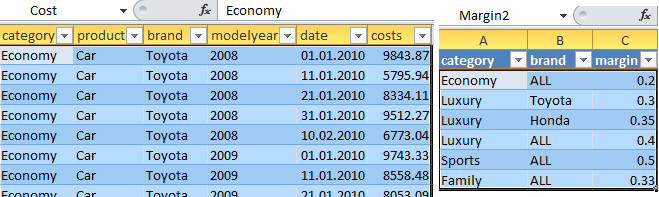You could obtain price table with table multiplication (see table function named Table Arithmetics) but in this example we will use the row transformer with a user-defined function (UDF).

First, combine cost and margin tables to obtain a table named Combined which includes both key figures costs and margin: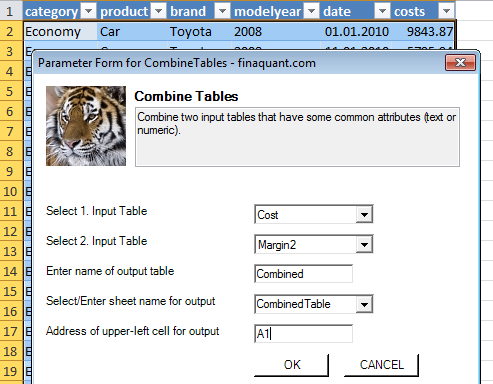An excerpt from the resultant table named Combined: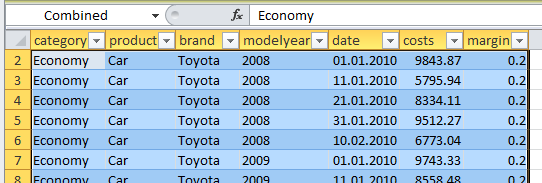Second, insert a new field (key figure) named price into this table. We will calculate the prices in the third step using the row transformer with UDF.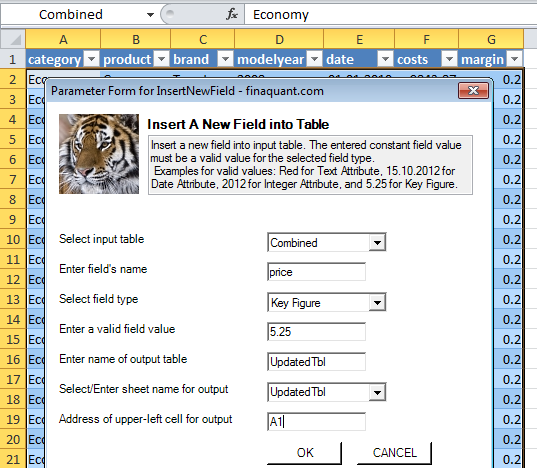An excerpt from the resultant table named UpdatedTbl including all the key figures costs, margin and price: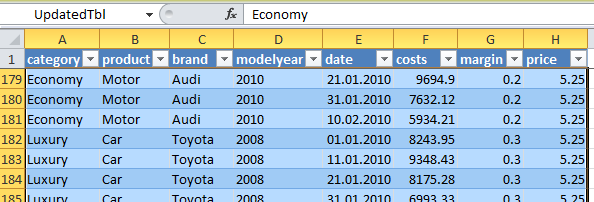Now that we have all the relevant fields in a table, we can finally calculate prices with the user-defined function:
`KF["price"] = KF["costs"] * (1 + KF["margin"]);`TA: Text Attribute
NA: Numeric Attribute (of type date or integer)
KF: Key Figure

In the example above, user-defined function is a simple formula:
`KF["price"] = KF["costs"] * (1 + KF["margin"]);`

As already mentioned, a user-defined function (UDF) can include anything including programming structures like if statements, provided that it is a valid C# code. Following code examples are all valid user-defined functions that apply on rows of PriceTbl:

1) Conditional Price Calculation:
Apply a fixed price margin 25% for the product category Economy:

```if (TA["category"] == "Economy") KF["price"] = 1.25 * KF["margin"]; else KF["price"] = KF["costs"] * (1 + KF["margin"]);```

2) Update category if margin is larger than 30%

`if (KF["margin"] > 0.3) TA["category"] = "Luxury";`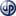Copyright secured by Digiprove © 2014 Tunc Ali Kütükcüoglu
This entry was posted in Calculation engine and tagged , . Bookmark the permalink.